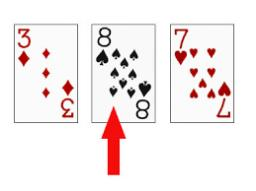# Increased 2843

Which number has the property that if we subtract from its five times increased by five and its three times reduced by 3, we get the original number again?

x =  -8

### Step-by-step explanation:

5x+5-(3x-3)=x

5·x+5-(3·x-3)=x

x = -8

x = -8/1 = -8

x = -8

Our simple equation calculator calculates it.Did you find an error or inaccuracy? Feel free to write us. Thank you!

Tips for related online calculators
Do you have a linear equation or system of equations and looking for its solution? Or do you have a quadratic equation?